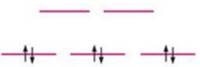Chapter 21, Problem 49E

Chapter
Section
Textbook Problem

# Match the crystal field diagrams given below with the following complex ions. Cr(NH3)5Cl2+ Co(NH3)4Br2+ (assume strong field) Fe(H2O)63+ (assume weak field) (a)(b)(c)(a)

Interpretation Introduction

Interpretation: The given crystal field diagrams are to be matched with the correct complex ions.

Concept introduction: Weak field ligand that has small crystal field splitting energy gives high spin due to presence of maximum unpaired electrons in lower energy t2g orbitals and higher energy orbitals.

Strong field ligand that has large crystal field splitting energy gives low spin due to the presence of maximum paired electrons in lower energy t2g orbitals.

To determine: If the given crystal field diagram corresponds to Cr(NH3)5Cl2+,Co(NH3)4Br2+ ion or Fe(H2O)63+ ion.

Explanation

The given crystal field diagram is,

Figure 1

The complex compound is Fe(H2O)63+ (Weak field).

Oxidation state of neutral Water (H2O) is 0.

It is assumed that the oxidation state of metal ion Iron (Fe) is x.

The given complex compound contains six water molecules. Since, the overall charge on the stable compound is +3

(b)

Interpretation Introduction

Interpretation: The given crystal field diagrams are to be matched with the correct complex ions.

Concept introduction: Weak field ligand that has small crystal field splitting energy gives high spin due to presence of maximum unpaired electrons in lower energy t2g orbitals and higher energy orbitals.

Strong field ligand that has large crystal field splitting energy gives low spin due to the presence of maximum paired electrons in lower energy t2g orbitals.

To determine: If the given crystal field diagram corresponds to Cr(NH3)5Cl2+,Co(NH3)4Br2+ ion or Fe(H2O)63+ ion.

(c)

Interpretation Introduction

Interpretation: The given crystal field diagrams are to be matched with the correct complex ions.

Concept introduction: Weak field ligand that has small crystal field splitting energy gives high spin due to presence of maximum unpaired electrons in lower energy t2g orbitals and higher energy orbitals.

Strong field ligand that has large crystal field splitting energy gives low spin due to the presence of maximum paired electrons in lower energy t2g orbitals.

To determine: If the given crystal field diagram corresponds to Cr(NH3)5Cl2+,Co(NH3)4Br2+ ion or Fe(H2O)63+ ion.

### Still sussing out bartleby?

Check out a sample textbook solution.

See a sample solution

#### The Solution to Your Study Problems

Bartleby provides explanations to thousands of textbook problems written by our experts, many with advanced degrees!

Get Started